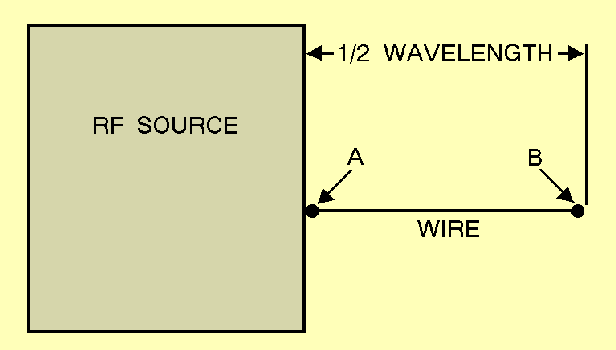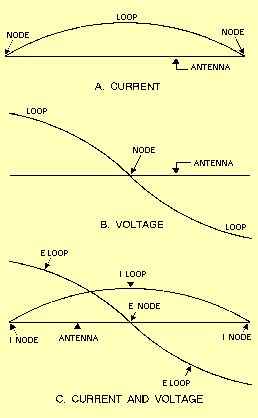Custom SearchThe electromagnetic radiation from an antenna is made up of two components, the E field and the H field. We discussed these fields in chapters 1 and 2. The two fields occur 90 degrees out of phase with each other. These fields add and produce a single electromagnetic field. The total energy in the radiated wave remains constant in space except for some absorption of energy by the Earth. However, as the wave advances, the energy spreads out over a greater area and, at any given point, decreases as the distance increases. Various factors in the antenna circuit affect the radiation of these waves. In figure 4-5, for example, if an alternating current is applied at the A end of the length of wire from A to B, the wave will travel along the wire until it reaches the B end. Since the B end is free, an open circuit exists and the wave cannot travel farther. This is a point of high impedance. The wave bounces back (reflects) from this point of high impedance and travels toward the starting point, where it is again reflected. The energy of the wave would be gradually dissipated by the resistance of the wire of this back-and-forth motion (oscillation); however, each time it reaches the starting point, the wave is reinforced by an amount sufficient to replace the energy lost. This results in continuous oscillations of energy along the wire and a high voltage at the A end of the wire. These oscillations are applied to the antenna at a rate equal to the frequency of the rf voltage. Figure 4-5. - Antenna and rf source.These impulses must be properly timed to sustain oscillations in the antenna. The rate at which the waves travel along the wire is constant at approximately 300,000,000 meters per second. The length of the antenna must be such that a wave will travel from one end to the other and back again during the period of 1 cycle of the rf voltage. Remember, the distance a wave travels during the period of 1 cycle is known as the wavelength and is found by dividing the rate of travel by the frequency. Look at the current and voltage (charge) distribution on the antenna in figure 4-6. A maximum movement of electrons is in the center of the antenna at all times; therefore, the center of the antenna is at a low impedance. This condition is called a STANDING WAVE of current. The points of high current and high voltage are known as current and voltage LOOPS. The points of minimum current and minimum voltage are known as current and voltage NODES. View A shows a current loop and current nodes. View B shows voltage loops and a voltage node. View C shows the resultant voltage and current loops and nodes. The presence of standing waves describes the condition of resonance in an antenna. At resonance the waves travel back and forth in the antenna reinforcing each other and the electromagnetic waves are transmitted into space at maximum radiation. When the antenna is not at resonance, the waves tend to cancel each other and lose energy in the form of heat. Figure 4-6. - Standing waves of voltage and current on an antenna.Q.4 If a wave travels exactly the length of an antenna from one end to the other and back during the period of 1 cycle, what is the length of the antenna?Q.5 What is the term used to identify the points of high current and high voltage on an antenna?Q.6 What is the term used to identify the points of minimum current and minimum voltage on an antenna?Integrated Publishing, Inc. - A (SDVOSB) Service Disabled Veteran Owned Small Business